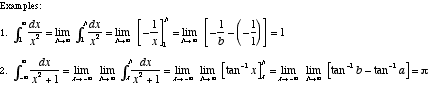index: click on a letter A B C D E F G H I J K L M N O P Q R S T U V W X Y Z A to Z index index: subject areas numbers & symbols sets, logic, proofs geometry algebra trigonometry advanced algebra & pre-calculus calculus advanced topics probability & statistics real world applications multimedia entrieswww.mathwords.com about mathwords website feedback

 Improper Integral A definite integral for which the integrand has a discontinuity between the bounds of integration, or which has ∞ and/or –∞ as a bound. Improper integrals are evaluated using limits as shown below. If the limit exists and is finite, we say the integral converges. If the limit does not exist or is infinite, we say the integral diverges.See also Integral test